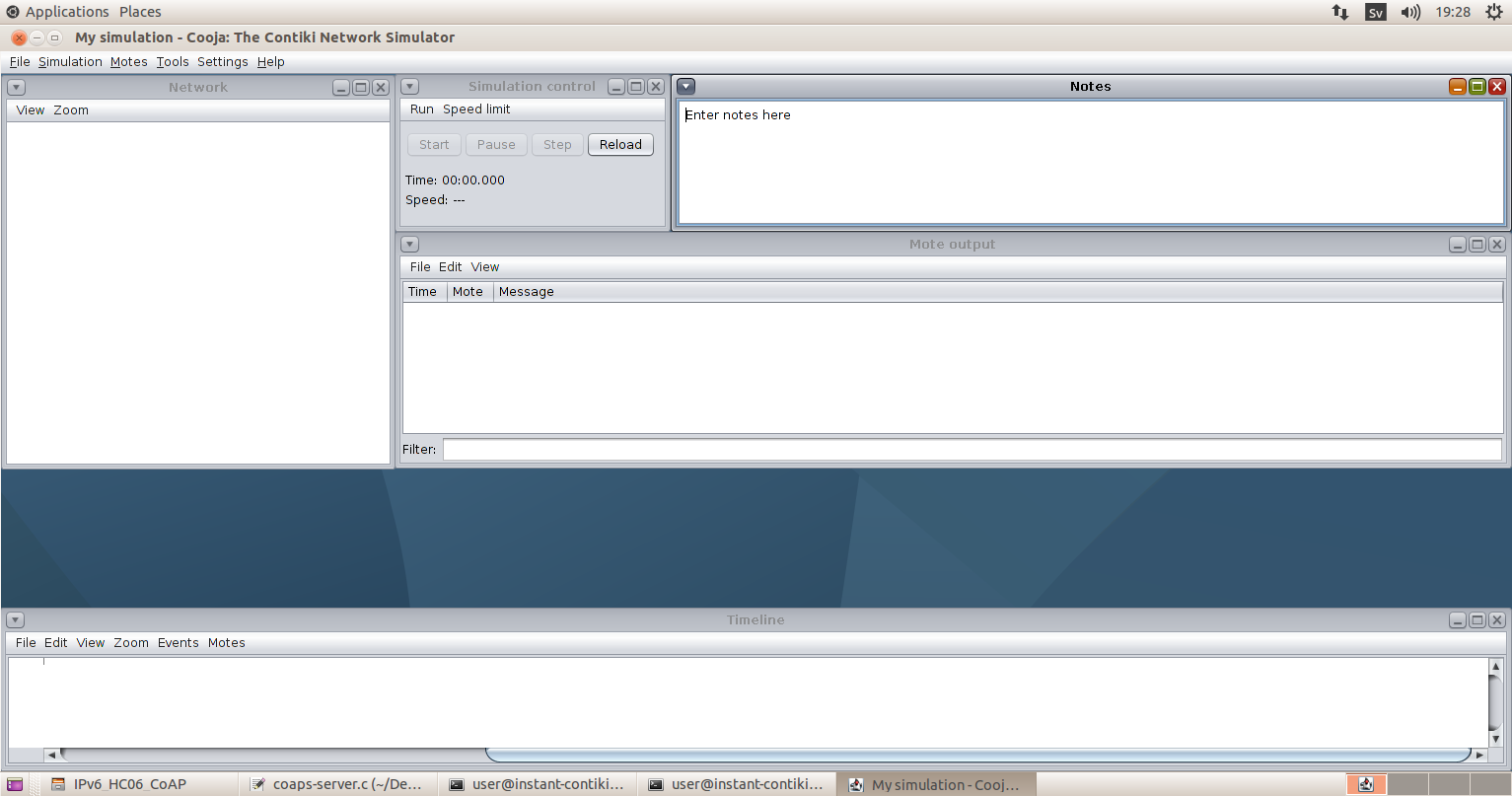• #5, First Floor, 4th Street Dr. Subbarayan Nagar Kodambakkam, Chennai-600 024 Landmark : Samiyar Madam
• pro@slogix.in
• +91- 81240 01111

#### Social List

##### How to calculate the Performance of DTLS for CoAP in Contiki Cooja Simulator?
###### Description

The packet delivery ratio is the ratio of packets successfully received to the total sent data packets.

Energy consumption is the amount of energy or power used to transmit or receive data packets.

###### Step-1

Open file to modify to calculate energy consumption at following location:

home/ contiki/ examples/ipv6/CoAP-IDs/IPv6_Hc06?CoAP_tinydtls/coaps-client.c

Edit and use following code inside a Response handler function

unsigned long cpu_time, lpm_time, rx_time, tx_time;

lpm_time = energest_type_time

(ENERGEST_TYPE_LPM);

cpu_time = energest_type_time

(ENERGEST_TYPE_CPU);

rx_time = energest_type_time

(ENERGEST_TYPE_LISTEN);

tx_time = energest_type_time

(ENERGEST_TYPE_TRANSMIT);

unsigned long energy_consumed;

unsigned long t1;

t1 = 1.8 * cpu_time ;

unsigned long t2;

t2 = 0.0545 * lpm_time;

unsigned long t3;

t3 = 20.0 * rx_time;

unsigned long t4;

t4=17.7*tx_time;

unsigned long t11;

unsigned long t22;

unsigned long t33;

t11 = (20.0 * rx_time + 17.7 * tx_time ) * 3 ;

t22 =  (unsigned long)

(((1.8 * cpu_time + 0.0545 * lpm_time + 20.0

* rx_time   + 17.7 Code: * tx_time ) * 3) /

RTIMER_SECOND);

t33 =  (unsigned long) (((t22 *

(1.8 * cpu_time + 0.0545 * lpm_time)) * 3)

/   RTIMER_SECOND);

power_consumed = (unsigned long)

(t11 / RTIMER_SECOND);

printf(“Energy CONSUMPTION IS %lu ID

%d\n”,t33, node_id); // take   performance

}###### Step-2

Open contiki cooja network simulator and create new simulation(ctrl+n).

rpl-border-router.c, er- example-server.c, er-example-client.c create motes for

Server ,client location: home/contiki/examples/ er-rest-example.

Border router location: home/contiki/examples/ipv6/ rpl-border-router

Connect the router to server .In terminal at above location.

make-connect-router-cooja

Code for to calculate energy consumption modify in existing (.csc) script file before re-simulation.###### Step-3

Performance

< script>TIMEOUT(300000, log.log("Performance Calculation" + "\n"));

packetsSent = new Array();

timeSent = new Array();

count = new Array();

nodeCount = 27;

data_length = 23;

senderID = 0;

PDR=0;

e_count = 0;

for(i = 3; i <= nodeCount; i++)

{

packetsSent[i] = 0;

timeSent[i] = 0;

}

while(1) {

YIELD();

msgArray = msg.split(' ');

if(msgArray.equals("Sending"))

{

if(msgArray.length == 5)

{

// sent packet

senderID = parseInt(msgArray);

packetsSent[senderID]++;

timeSent[senderID] = time;

}

}

if(msgArray.equals("Got"))

{

if(msgArray.length == 6)

{

{

}

totalclient=0;

for(i = 3; i <= nodeCount; i++)

{

totalclient++;

totalSent += packetsSent[i];

log.log("MoteID= " + i + " ReceivedPackets= " + packetsReceived[i] + " SendingPackets= " + packetsSent[i] + "\n");

}

log.log(" Generated Packets " + totalSent + "\n");

log.log("Packet Delivery Ratio" + PDR + "\n");

if(msgArray.equals("Data")) {

if(msgArray.length == 6) {

e_count++;

energy_t = parseInt(msgArray);

totalenergy = energy_t + totalenergy;

energy_consumption = totalenergy / e_count;

// now unit in milliwatts/ticks= 1milliwats=0.001 joules

energyconsumption = energy_consumption / 1000;

energyconsumption = energyconsumption / 128;

log.log(" EnergyConsumption = " + energy_consumption + " EnergyConsumption (Joules) = " + energyconsumption + "e_count" + e_count + "\n");

}

} }